# Sanscript

Typing discipline:
Versions and implementations (Collapse all | Expand all):
Programming language

Sanscript is a visual dataflow programming language, available via its native IDE. It was developed by Northwoods Software as a simple visual scripting language. Its main purpose is manipulating file system, interacting with other applications and performing Dynamic Data Exchange (DDE) functions, so it provides a large set of library functions for these purposes. Sanscript works only with Windows platform.

Sanscript IDE is divided in three main parts: Overview, Catalog and Canvas.

Overview window (on the left) displays the list of folders available in Sanscript. These folders are grouped into General (libraries of standard functions), Examples and Templates (groups of ready-to-run sample programs and sample functions provided in the distribution) and USER (user-defined programs).

Catalog window (on the bottom) displays the contents of chosen folder: functions and programs. Functions can be dragged into Canvas for further usage, and programs can be clicked for editing. Note that a function can be not only library one but also a user-defined one.

Canvas window (the main one) displays the flowgram to be edited.

Elementary program unit in Sanscript is a function — an entity which accepts some input, processes it and turns it into some output. A function is displayed as a block with an image which denotes its purpose and a name which can (optionally) be changed to a more descriptive one. This block has inlets and outlets — slots which mark input and output data flows of the function and are used to connect the function to other blocks of the flowgram with links.

Some functions have only outlets: these are used to generate input data of the program. The most popular example of such functions is “Constant” (used to hold some predefined constant). Even “Prompt” function, which asks the user to enter some value, has an inlet for setting the text of prompt. In flowgrams of functions, the inlets of the function are represented with blocks of special types.

Some functions have only inlets: these are used mostly for outputting the results of calculation, like “Display Message”.

Most flow control statements in other languages have their function equivalent in Sanscript. Thus, loops are done with “Repeat” function (break statement is called “Stop Repeat”); `if-then-else` and `case` statements are replaced with “Pick One”.

Sanscript provides simple data types: integer, decimal (floating-point number), text (equivalent of string data types in other languages), boolean and pathname. Besides, there are two complex data types — List and Record, along with a number of functions to process them.

## Examples:

Example for versions Sanscript 2.2

Sanscript is a fully visual programming language, so no source code is available. See screeshots instead.

This example contains a lot of branches on condition, and in Sanscript each branch is described in a new flowgram.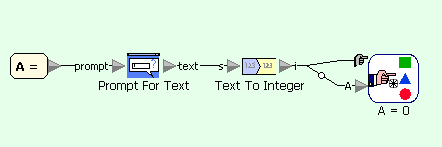Quadratic equation example in Sanscript (main flowgram)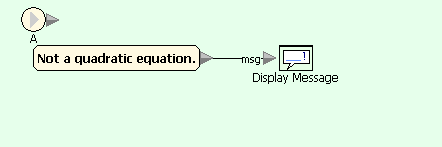Quadratic equation example in Sanscript (on condition A=0)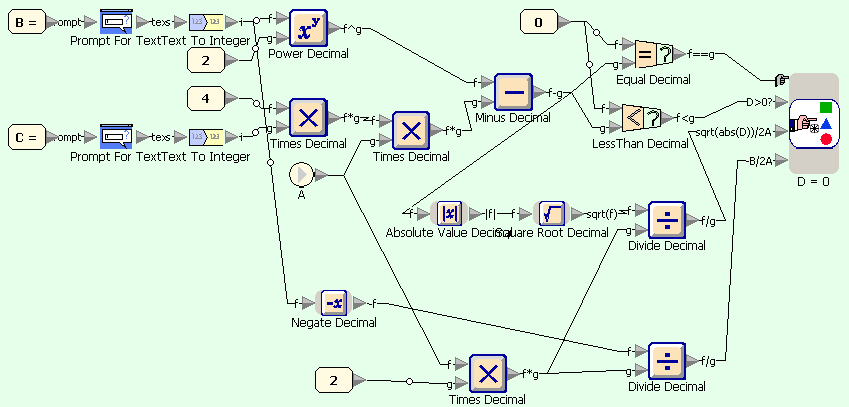Quadratic equation example in Sanscript (on condition A=default)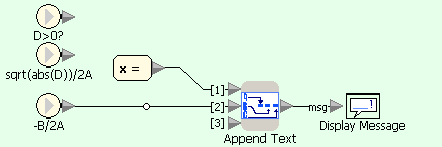Quadratic equation example in Sanscript (on condition D=0)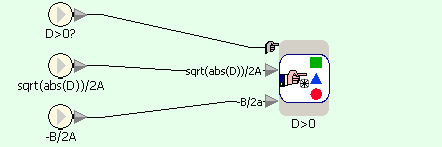Quadratic equation example in Sanscript (on condition D=default)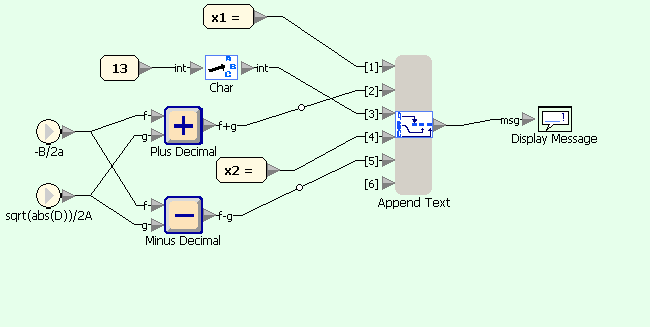Quadratic equation example in Sanscript (on condition D>0)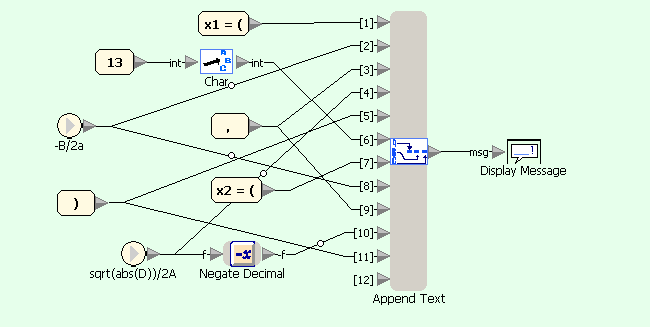Quadratic equation example in Sanscript (on condition D<0)

### Fibonacci numbers:

Example for versions Sanscript 2.2

Sanscript is a fully visual programming language, so no source code is available. See screeshots instead.

Fibonacci numbers are calculated in the same way as factorial: a loop calculates a list of numbers, starting with two first ones, and then this list is concatenated to produce the output. Within the loop current number is added to the list and replaced with next number, while next number is replaced with a sum of current and next numbers.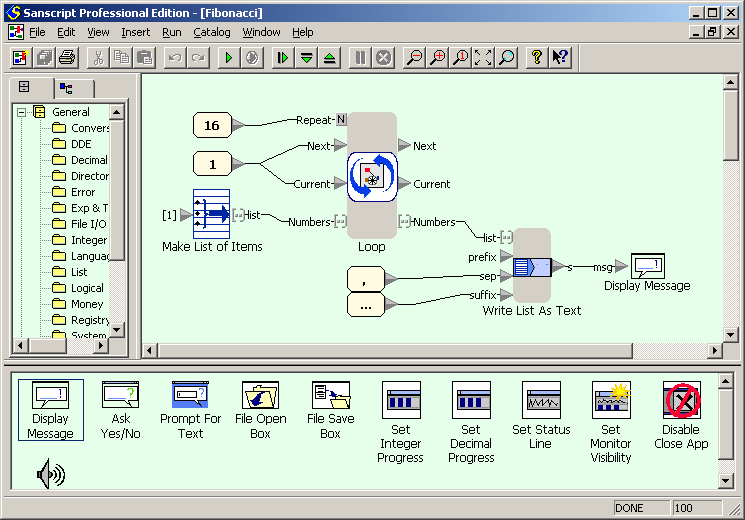Fibonacci numbers example in Sanscript (flowgram)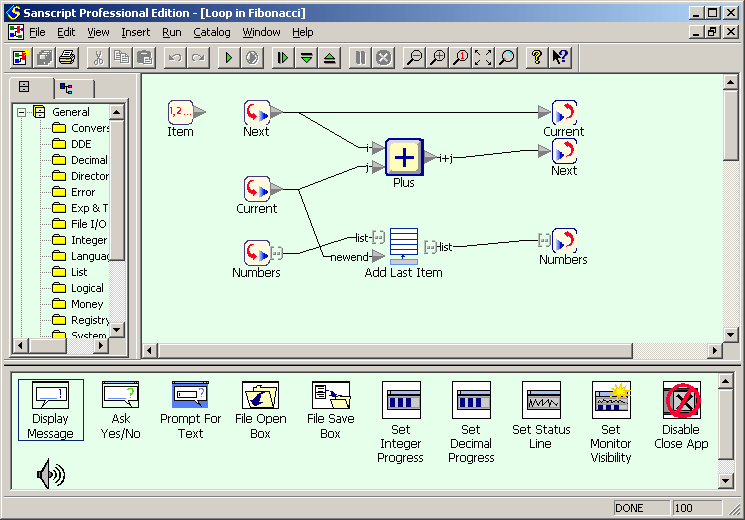Fibonacci numbers example in Sanscript (repeat)

### Factorial:

Example for versions Sanscript 2.2

Sanscript is a fully visual programming language, so no source code is available. See screeshots instead.

First screenshot shows the flowgram of the example. The main part of it is “Repeat” function (an analogue of loop in other languages) which calculates the factorials of numbers from 1 to 16 and stores them in a list. This function has two inlet/outlet pairs which correspond to global variables in other languages: `factorial` and `message`. `factorial` is an integer which stores the current value of factorial. `message` is a list of text lines `n! = (n!)`. The “Repeat” function has one more inlet `N` which defines the number of iterations to be done. The inlets are initialized with constants and an empty list, and the loop starts. Once the calculations are done, `message` has to be displayed. This can be done using `Write List as Text` function, which writes all elements of list into a single text variable. The separator between elements of the list is defined with `sep` inlet; in this case it is newline character, generated using “Char” function which converts an ASCII-code into a corresponding character. Finally, the concatenated list is displayed using “Display Message” function.

Second screenshot shows the intrinsics of “Repeat” function. Element marked with “1, 2 …” is loop counter, started with 1 and incremented by 1 each iteration. On each iteration `factorial` is multiplied by the value of loop counter (using “Times” function). After this its new value is concatenated with loop counter and “! = ” constant string (using “Append Text” function) to produce one line of output. Finally, this line is added to the list `message` (using “Add Last Item”) function.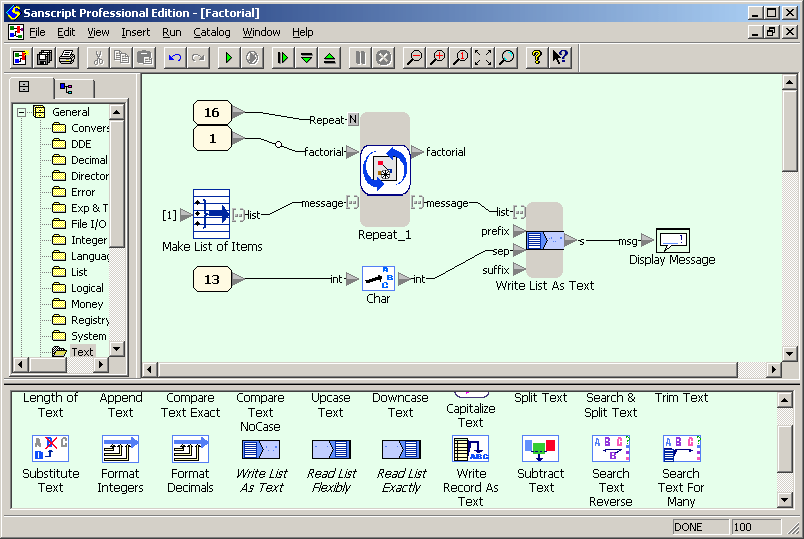Factorial example in Sanscript (flowgram)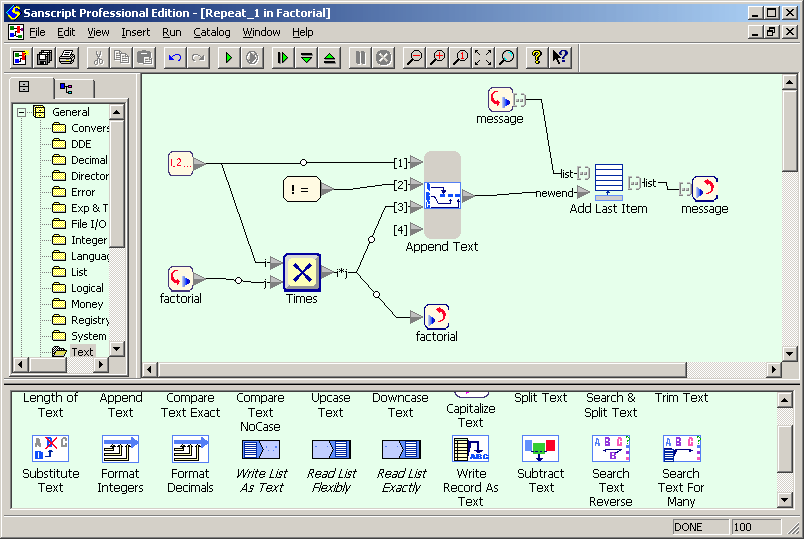Factorial example in Sanscript (repeat)

### Hello, World!:

Example for versions Sanscript 2.2

Sanscript is a fully visual programming language, so no source code is available. See screenshot instead.

The flowgram (Sanscript equivalent of program) for this example consists of two functions: constant “Hello, World!” and function “Display Message”. Once the flowgram runs, the constant function produces the message on its outlet. An arrow link connects this outlet to inlet of “Display Message” function, so it creates a pop-up window with this message.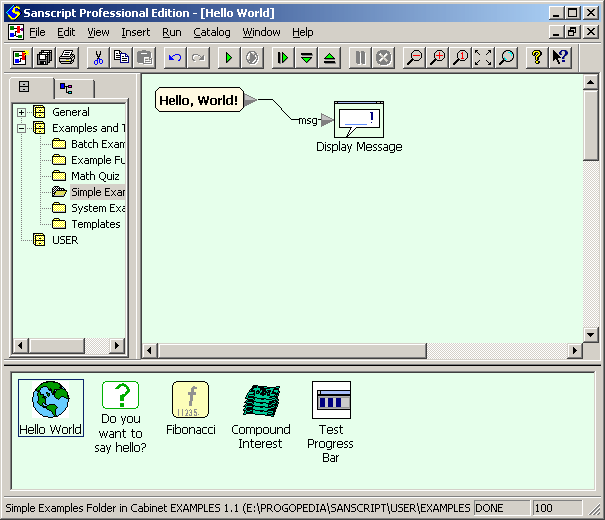"Hello, World!" example in Sanscript

### CamelCase:

Example for versions Sanscript 2.2

First flowgram converts user-entered text in lowercase and passes it into a character-wise processing loop. Second diagram uses `Split Text` block for extracting next character of the string, which is then compared with characters with ASCII-codes before a and after z, and the result of comparison is passed to selection block. Third diagram shows processing of letters: depending on the value of `lastSpace` either the letter or its uppercase equivalent are appended to the result, and `lastSpace` is set to `FALSE`. Fourth diagram shows processing of non-letter characters: it only sets the value of `lastSpace` to `TRUE`.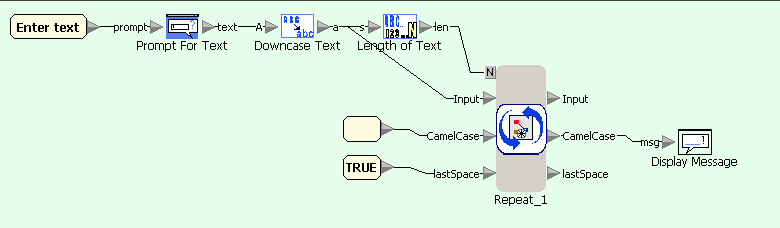CamelCase example in Sanscript (main flowgram)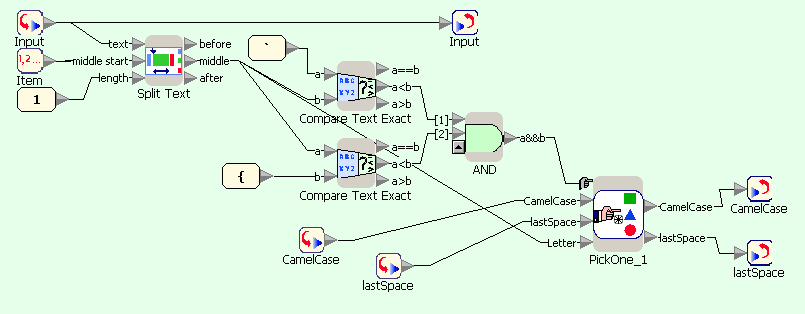CamelCase example in Sanscript (repeat block)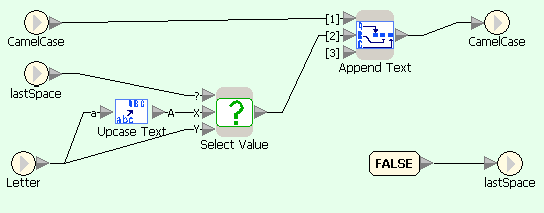CamelCase example in Sanscript (if character is letter)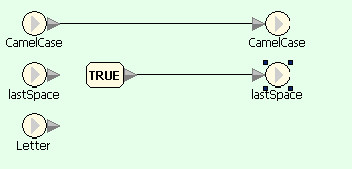CamelCase example in Sanscript (if character is not a letter)